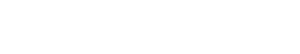# Perplexity

Perplexity is a measure of how well a probability model predicts a sample. It is often used in the text analytics industry to compare the goodness of fit of different probability models. For example, if you were training a language model on a corpus of English text, you could evaluate the perplexity of the model on a held-out set of text. A lower perplexity would indicate a better fit.

Perplexity is defined as:

perplexity = 2 ^ {-log P(w)}

Where:

P(w) is the probability of the word w

log is the natural logarithm

Perplexity can be thought of as a measure of how surprises or unexpected an event is. For example, if you were to flip a fair coin, you would expect it to come up heads 50% of the time. This would give a perplexity of 2 ^ {-log (0.5)} = 2. If the coin came up heads 100% of the time, the perplexity would be 2 ^ {-log (1.0)} = 1. If the coin came up heads 0% of the time, the perplexity would be 2 ^ {-log (0.0)} = infinity.

There are many ways to calculate perplexity, but the most common way is to use the natural logarithm.

## Importance of Perplexity

The data scientist should develop a perplexity metric. It’s important to recognize that perplexity can be used to evaluate the goodness of fit of different probability models. A perplexity is also a useful tool if you want to compare the predictive power of different models. For example, you may have two language models that both have a perplexity of 100 on the training data. However, if model A has a perplexity of 50 on the held-out data, and model B has a perplexity of 150, then model A is better at generalizing from the training data to unseen data.

Perplexity can also be used to compare different probability models for the same phenomenon. For example, you may want to compare a trigram language model with a bigram language model. If the trigram model has a lower perplexity, then it is a better model for the task at hand.

There are many factors that go into building a good probability model, and perplexity is just one metric that data scientists should consider. However, it is an important metric, and data scientists should be familiar with how to calculate it and what it means.Unlock the power of actionable insights with AI-based natural language processing.